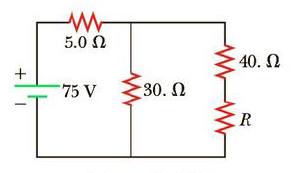Chapter 18, Problem 58AP

Chapter
Section
Textbook Problem

The resistor R in Figure P18.58 dissipates 20 W of power. Determine the value of R.Figure P18.58

(a)

To determine
the graph of power dissipated in the load resistor as a function of the load resistance.

Explanation

Given Info:

Internal resistance of the battery is 10.0Ω .

The open circuit voltage of the battery is 12.0V .

The variable resistance connected across the battery is, 0 to 30.0Ω .

Explanation:

Thus, from the circuit; the current in the circuit is,

I=12.0VR+10.0Ω (I)

Formula to calculate the power delivered to the load resistor is,

• I is the current in the circuit
• R is the load resistor

Substitute equation (I) in (II),

(b)

To determine

To demonstrate: the theorem “The power delivered to a load is a maximum if the load resistance equals the internal resistance of the source”.

Still sussing out bartleby?

Check out a sample textbook solution.

See a sample solution

The Solution to Your Study Problems

Bartleby provides explanations to thousands of textbook problems written by our experts, many with advanced degrees!

Get Started

Find more solutions based on key concepts